Systems of Linear Equations: Definitions (page 1 of 7)

Sections: Definitions, Solving by graphing, Substitition, Elimination/addition, Gaussian elimination.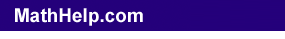A "system" of equations is a set or collection of equations that you deal with all together at once. Linear equations (ones that graph as straight lines) are simpler than non-linear equations, and the simplest linear system is one with two equations and two variables.

Think back to linear equations. For instance, consider the linear equation y = 3x – 5. A "solution" to this equation was any x, y-point that "worked" in the equation. So (2, 1) was a solution because, plugging in 2 for x:

3x – 5 = 3(2) – 5 = 6 – 5 = 1 = y

On the other hand, (1, 2) was not a solution, because, plugging in 1 for x:

3x – 5 = 3(1) – 5 = 3 – 5 = –2

...which did not equal y (which was 2, for this point). Of course, in practical terms, you did not find solutions to an equation by picking random points, plugging them in, and checking to see if they "work" in the equation. Instead, you picked x-values and then calculated the corresponding y-values. And you used this same procedure to graph the equation. This points out an important fact: Every point on the graph was a solution to the equation, and any solution to the equation was a point on the graph.

Now consider the following two-variable system of linear equations:

y = 3x – 2
y = –x – 6

 Since the two equations above are in a system, we deal with them together at the same time. In particular, we can graph them together on the same axis system, like this: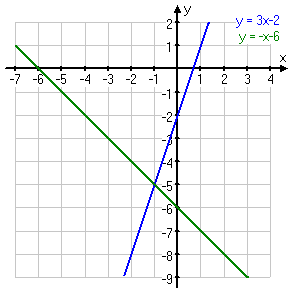A solution for a single equation is any point that lies on the line for that equation. A solution for a system of equations is any point that lies on each line in the system. For example, the red point at right is not a solution to the system, because it is not on either line: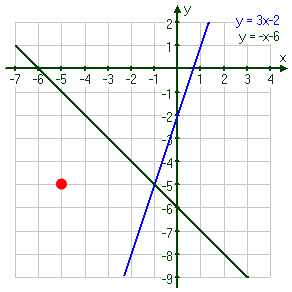The blue point at right is not a solution to the system, because it lies on only one of the lines, not on both of them: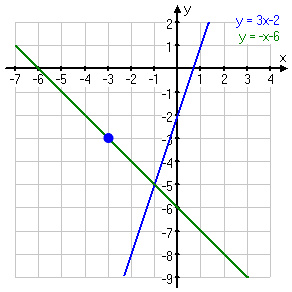The purple point at right is a solution to the system, because it lies on both of the lines: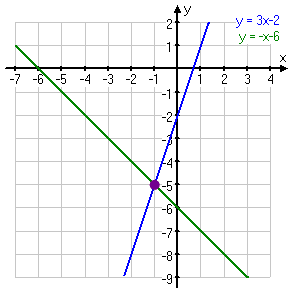In particular, this purple point marks the intersection of the two lines. Since this point is on both lines, it thus solves both equations, so it solves the entire system of equation. And this relationship is always true: For systems of equations, "solutions" are "intersections". You can confirm the solution by plugging it into the system of equations, and confirming that the solution works in each equation.

Content Continues Below

• Determine whether either of the points (–1, –5) and (0, –2) is a solution to the given system of equations.
• y = 3x – 2
y = –x – 6

To check the given possible solutions, I just plug the x- and y-coordinates into the equations, and check to see if they work.   Copyright © Elizabeth Stapel 2003-2011 All Rights Reserved

checking (–1, –5):

(–5) ?=? 3(–1) – 2
–5 ?=? –3 – 2
–5 = –5
(solution checks)

(–5) ?=? –(–1) – 6
–5 ?=? 1 – 6
–5 = –5
(solution checks)

Since the given point works in each equation, it is a solution to the system. Now I'll check the other point (which we already know, from looking at the graph, is not a solution):

checking (0, –2):

(–2) ?=? 3(0) – 2
–2 ?=? 0 – 2
–2 = –2
(solution checks)

So the solution works in one of the equations. But to solve the system, it has to work in both equations. Continuing the check:

(–2) ?=? –(0) – 6
–2 ?=? 0 – 6
–2 ?=? –6

But –2 does not equal –6, so this "solution" does not check. Then the answer is:

only the point (–1, –5) is a solution to the system

Top  |  1 | 2 | 3 | 4 | 5 | 6 | 7  |  Return to Index  Next >>

 Cite this article as: Stapel, Elizabeth. "Systems of Linear Equations: Definitions." Purplemath. Available from     https://www.purplemath.com/modules/systlin1.htm. Accessed [Date] [Month] 2016

MathHelp.com Courses

Purplemath:

Study Skills Survey